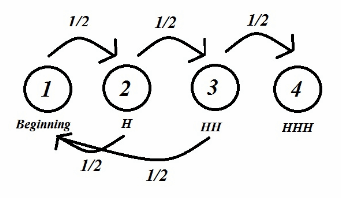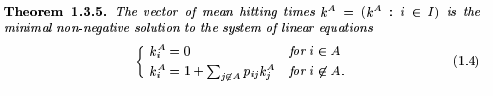#### 不停抛掷硬币直至连续3次出现正面，此时抛硬币的次数的期望是多少？

数学 随机过程 趣味数学    浏览次数：8318        分享

0yangyang   2018-09-05 12:54

##### 2个回答
5

$(1-p)(x+1)+p(1-p)(x+2)+p^2(1-p)(x+3)+3p^3=x$

2. 根据 http://www.aquatutoring.org/ExpectedValueMarkovChains.pdf 的 Example 7 可直接得期望为 14。

SofaSofa数据科学社区DS面试题库 DS面经lpq29743   2018-09-11 20:57

4$$P=\begin{bmatrix}0.5 & 0.5 & 0 & 0\\0.5 & 0 & 0.5 & 0 \\ 0.5 & 0 & 0 & 0.5\\ 0 & 0 & 0 & 1\end{bmatrix}$$

$x_{t+1}=x_{t} P$$K_i^A$是状态i到状态A的步数的期望

$K_1^4 = 1 + \sum\limits_{j = 1,2,3} {{P_{1j}}K_j^4} =1+0.5K_1^4+0.5K_2^4$

$K_2^4 = 1 + \sum\limits_{j = 1,2,3} {{P_{2j}}K_j^4} =1+0.5K_1^4+0.5K_3^4$

$K_3^4 = 1 + \sum\limits_{j = 1,2,3} {{P_{3j}}K_j^4} =1+0.5K_1^4$

SofaSofa数据科学社区DS面试题库 DS面经Zealing   2018-09-05 13:58相关主题